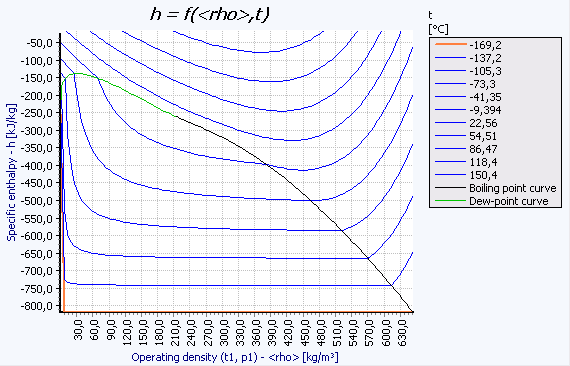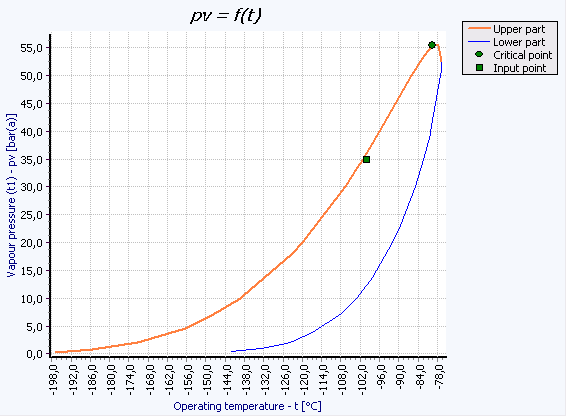# CONVAL FLUID PROPERTIES

### Fluid Properties

CONVAL® can calculate pressure and temperature-dependent properties according to various methods.
Almost all the program modules require a certain amount of information about the pressure and temperature-dependent properties of the medium being used in the process.
CONVAL® is capable of calculating this process data automatically for a large number of media (approx. 1000) in connection with the calculation program.
Three different calculation methods for process data are offered at present, namely:
1) Using approximation equations based on a property database for physical constants.
2) By interpolation on the basis of a property database for actual measurement series.
3) With the help of extremely accurate thermodynamic equations of state.

### Interpolation

If neither properties for approximation equations nor special calculation methods such as the steam table (IAPWS 97) for water are available, CONVAL® can interpolate the information it needs on the basis of measured values.
CONVAL® integrates an extensible property database containing around 200 substances (thermal oils, for instance), for which operating data is available in the form of measured values.
Curves of the operating density, dynamic viscosity, boiling temperature, specific heat capacity, thermal conductivity and isentropic exponent can be entered here for liquids.
Curves of the specific heat capacity, thermal conductivity and isentropic exponent can be entered additionally for gases.

### RUB for CONVAL

The latest RUB release adds nine new media to the already long list of available substances and includes options for calculating mixtures according to the GERG 2004 reference equation of state and creating phase diagrams.
Precise determination of the properties at the operating points provides the foundation for reliable sizing and calculation in all CONVAL modules.
The Thermodynamics Module 1.0 introduced with CONVAL 6.0 enables an extremely precise calculation of the thermodynamic and transport properties of selected substances in a very wide pressure-temperature range to be taken as a sizing basis. The thermodynamic properties are derived from highly accurate equations of state, many of which are the acknowledged international standard. The transport properties are calculated using the best equations published in this field.
The new Thermodynamics Module 2.0 additionally integrates functionalities for calculating and visualizing the thermophysical properties of selected fluids.
The 64 media already supported by Release 1.0 of the Thermodynamics Module have now been augmented with nine new substances:
Acetone
cis-2-Butene,
Ethanol
Freon 116
Freon 227ea
Isobutene
Carbon oxysulfide
Sulfur dioxide
Trans-2-Butene.
By specifying any combination of two property values out of temperature T, pressure p, density ρ, specific entropy s, specific enthalpy h, and steam quality, it is possible to calculate a large number of thermophysical properties as a function of the input data.
Isolines of the thermodynamic properties temperature T, pressure p, density ρ, specific enthalpy h, specific entropy s, and steam quality x can be calculated and printed out in the form of tables. All thermophysical properties included in the program module can be calculated along these isolines.### GERG-2004 XT08

By implementing GERG-2004 XT08, CONVAL gains access to a host of new applications, for instance in LNG (Liquefied Natural Gas) projects. The AGA-8 equation so far available since CONVAL 7.0 only covers the gaseous state of a very limited spectrum of natural gas mixtures.
The GERG-2004 XT08 equation that has been established at the Chair of Thermodynamics of the Ruhr-Universität Bochum (Prof. Dr.-Ing. W. Wagner) describes thermodynamic properties in the gaseous, liquid, hypercritical, and gas-liquid equilibrium states. It can be used to calculate the thermodynamic properties of gaseous and liquefied natural gases, a wide range of other compositions, and binary mixtures. The mixture calculation is integrated in all calculation modules, such as control valves. The phase equilibrium can be represented in the Thermodynamics Module as a pv(t) diagram of the mixture.
The graph above shows the phase equilibrium of a typical natural gas
Components of natural gases taken into account in the GERG-2004 XT08 equation of state.
Methane
Nitrogen
Carbon dioxide
Ethane
Propane
n-Butane
Isobutane
n-Pentane
Isopentane
n-Hexane
n-Heptane
n-Octane
n-Nonane
n-Decane
Hydrogen
Oxygen
Carbon monoxide
Water
Hydrogen sulphide
Helium
Argon### Approximation

Approximation is a very useful option if no special calculation methods, such as the steam table (IAPWS 97) for water, are available.
CONVAL® integrates an extensible property database containing around 800 substances and their physical constants, which can be taken as a basis for pressure and temperature-dependent calculations of the substance properties.
The boiling pressure pv of a liquid can be calculated, for example, in its state at a temperature t1, or the boiling temperature tv of this liquid in its state at a pressure p1, in accordance with Riedel's correlation (error < 10%).
Alternatively, it is possible to calculate the real gas factors Z1 and Zn, and thus also the specific gas densities. This calculation is based on Newton and Raphson's correlations and on publications by Dranchuk, Purvis, Robinson and Takacs.
The operating density of liquids is calculated with the help of the physical constants stored in the database according to mathematical approximation methods described by R. W. Hankinson and G. H. Thomson. In conjunction with other authors these methods are also referred to as the COSTALD correlation. The error is less than 1% for the majority of applications and only exceeds 5% under a very limited number of boundary conditions.
Approximation methods are also available for calculating the isentropic exponent, the sound velocity, etc.